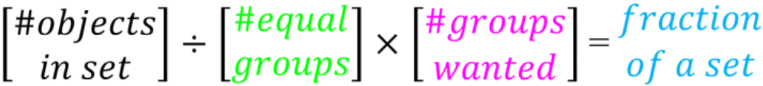# Fractions of a set#### All in One Place

Everything you need for better grades in university, high school and elementary.#### Learn with Ease

Made in Canada with help for all provincial curriculums, so you can study in confidence.#### Instant and Unlimited Help

0/1
##### Intros
###### Lessons
1. Introduction to Fractions of a Set:
How to find the fraction of a set of objects
0/11
##### Examples
###### Lessons
1. Picture models for fractions of a set
Picture models for fractions of a set
1. $\large \frac{1}{2}$ of 10 is ____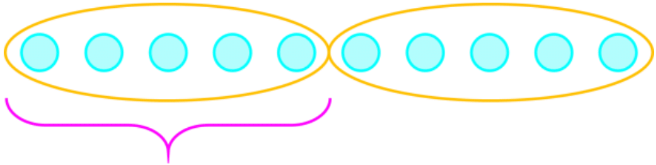2. ___ of 9 is 3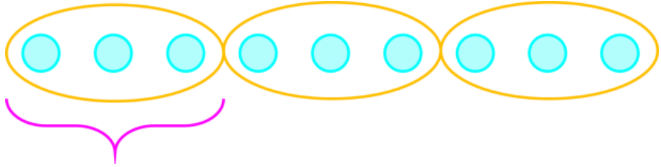2. Grouping circles for fractions of a set
For the set of circles, answer the sentence equation by:
• Drawing circles to make equal groups
• And then drawing a box around the given amount
1. $\large \frac{2}{3}$ of 12 is ____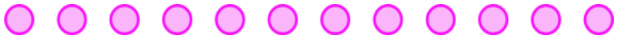2. $\large \frac{4}{5}$ of 15 is ____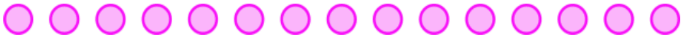3. Fractions of a set with division
Find fraction of the set and write as a division statement.
1. $\large \frac{1}{4}$ of 12 is ____
____ ÷ ____ = ____
2. $\large \frac{1}{2}$ of 18 is ____
____ ÷ ____ = ____
4. Fractions of a set with multiplication and division
Find fraction of the set and write a statement with both division and multiplication.
1. $\large \frac{2}{3}$ of 15 is ____
____ ÷ ____ × ____ = ____
2. $\large \frac{5}{6}$ of 24 is ____
____ ÷ ____ × ____ = ____
5. Word problems for fractions of a set
1. Raine has 20 socks. $\large \frac{3}{10}$ of the socks are yellow. How many socks are yellow?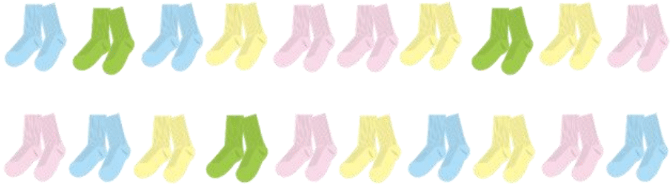2. 11 children are on the playground. $\large \frac{6}{11}$ of the children are boys. How many girls are on the playground?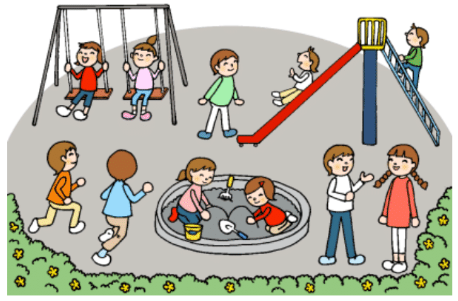3. Eli has 21 marbles. $\large \frac{2}{7}$ of the marbles are blue. How many not blue marbles does Eli have?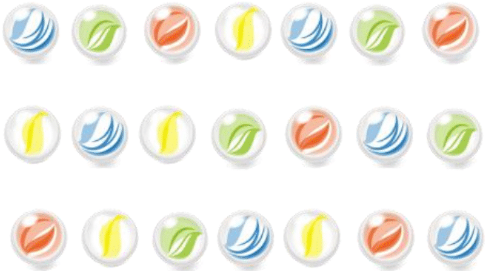0%
##### Practice
###### Topic Notes

In this lesson, we will learn:

• How to use fractions to talk about sets of objects
• How to use division and multiplication to solve for fraction of a set

Notes:

• We can use fractions to talk about sets of objects. We can use images or just simplify to a simple shape like a circle.
• Ex. If Austin has 8 jellybeans and he wants to give $\large \frac{1}{4}$ to her, how many jellybeans will he give?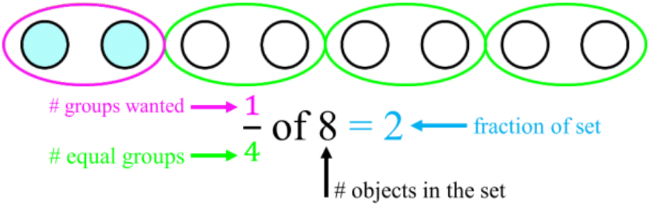• By making 4 equal groups and then giving just 1 of those groups, we realize that there is 2 in each group, so he gave his sister 2 jellybeans.
• We can use division and multiplication to find the fraction of a set
• Ex. After giving his sister jellybeans, Austin has $\large \frac{3}{4}$ of his 8 jellybeans left.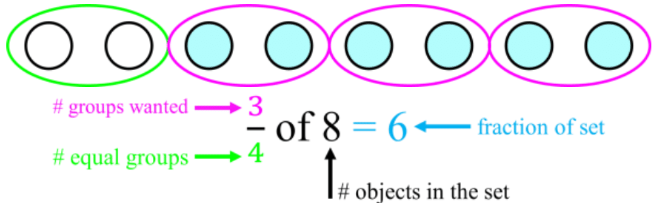• Step 1: divide 8 into 4 equal groups $\, \longrightarrow \,$ 8 ÷ 4 = 2
• Step 2: take the result and multiply by 3 groups $\, \longrightarrow \,$ 2 × 3 = 6
• The formula for a fraction of a set:
• following the format: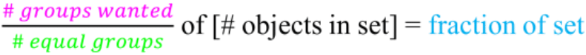• divide the denominator and multiply the numerator